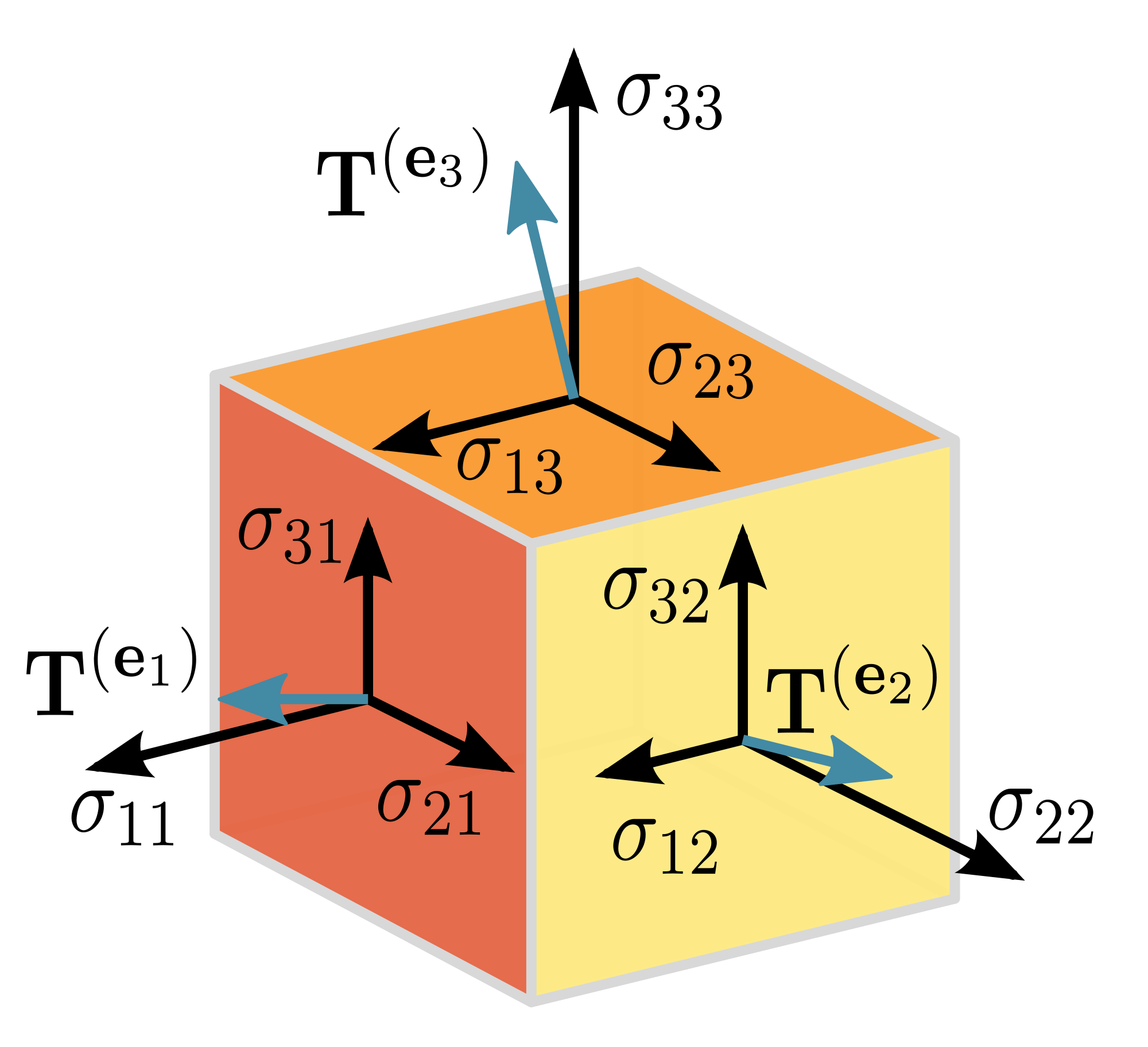# Tensorflow 5: Variables

My previous post. .  All my code can be found on github (5_Variables.ipynb)

#### Variables

We use variables to store data during computation.

#### Types

There are many different ways to create Variables.  Here are some examples.

```#Different Tensor Variables

# Basic
y = tf.Variable(10, name='y')

#array
array = tf.Variable([1,2,3,4], name='array')

# Zeros
zeros=tf.zeros([3,2])

# Ones
ones = tf.ones([3,2])

# Random uniform values
rand_uniform = tf.random_uniform([3,2], minval = -10, maxval=10)

# Normally distributed numbers
rand_normal = tf.random_normal([3,2],mean=0.0,stddev=3.0)```

We need to allocate memory for the variables.  We do that by calling

```# Add an op to initialize the variables.
init_op = tf.global_variables_initializer()

#execute with examples
session = tf.Session()
session.run(init_op)```

Then when the above commands are run we get:#### Changing values

To change the value of a variable we use the assign operation.

We can also save an operation on a variable and run it later.

```my_variable = tf.Variable(0)

increment_my_variable = my_variable.assign(my_variable + 1)```

Here i have created a new variable my_variable and initialized it to 0.

I have also created an operation called increment_my_variable that adds one to its current vale.

I can run both the operation or assign the variable directly.Above each time increment_my_variable runs it increments the value.  But we can also directly assign a value to my_variable as well.

#### Saving

If you want to save the values of variables use the tf.train.Saver() operation.

```# Use this operation to save the session variables
saver = tf.train.Saver()

# Run the save operation
save_path = saver.save(session, "./model.ckpt")
print("Saved: %s" % save_path)```This will create the files in the current directory.You will use this later to save the weights and biases of trained models.

#### Restoring

Then later call

`saver.restore(session, "./model.ckpt")`

To restore the values from the file.  Example: